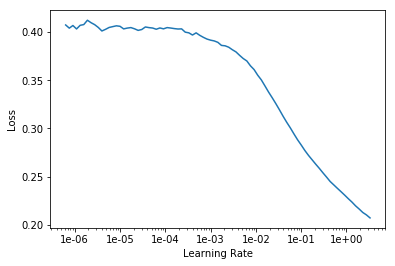# Tabular regressor unable to predict perfect linear relationship

Hi everyone, and thanks for all the work you put into this.

I am trying to do regression with the TabularList model. I have it working in the sense that it seems to train on data, validate and make predictions; however, it was giving very strange predictions. So to debug it, I reduced the model have one output label (called `velocity`) and one input feature (called `velocity_2`) which is identical to the output. Still the model gives wrong predictions, even though it’s a perfectly linear relationship.

Examinemus!

First a look at the input data.

``````print('Train shape:', train_df.shape)
print('Train tail:\n', train_df.tail())
print('Train correlations:\n', train_df.corr())
``````

gives

``````Train shape: (29925, 2)

velocity  velocity_2
0  0.574803    0.574803
1  0.574803    0.574803
2  0.574803    0.574803
3  0.574803    0.574803
4  0.574803    0.574803

Train tail:
velocity  velocity_2
4341  0.590551    0.590551
4342  0.354331    0.354331
4343  0.511811    0.511811
4344  0.527559    0.527559
4345  0.307087    0.307087

Train correlations:
velocity  velocity_2
velocity         1.0         1.0
velocity_2       1.0         1.0
``````

As expected the correlations are perfectly linear. Then I create and train the model:

``````# Split data into train and validate sets.
valid_idx = range(len(midi_df) - len(validate_df), len(midi_df))

continuous_names = ['velocity_2']
dep_var = 'velocity'
y_range = range(-1, 1)

procs = [Categorify, Normalize]
data = (TabularList.from_df(midi_df, path=data_folder, cat_names=[], cont_names=continuous_names, procs=procs)
.split_by_idx(valid_idx)
.label_from_df(cols=dep_var, label_cls=FloatList)
.databunch())

learn = tabular_learner(data, layers=[200, 100], emb_szs={}, y_range=y_range, metrics=exp_rmspe)

learn.fit_one_cycle(1, 1e-2)
``````

giving me

``````epoch     train_loss  valid_loss  exp_rmspe
1         0.053190    0.032207    0.123913
``````

I can also make predictions:

``````predictions, targets = learn.get_preds(DatasetType.Valid)
print('Predictions: ', predictions)
print('Expected: ', targets)
``````

giving me

``````Predictions:  tensor([[-5.1767e-01],
[-5.1767e-01],
[-4.1593e-01],
...,
[-2.0785e-03],
[-4.3088e-04],
[-3.8314e-04]])
Expected:  tensor([-0.4961, -0.4961, -0.4016,  ...,  0.1181,  0.2598,  0.2756])
``````

But surely with 30k samples of a perfectly linear relationship it should have a near-zero loss? I can only assume I am doing something wrong, but cannot figure out what, precisely. That’s where you come in! Any and all help would be much appreciated.

I’m using fastai v1.0.37.

``````\$ pip3 list | grep fastai
fastai              1.0.37
``````

Thanks!

1 Like

Maybe the learning rate is too big? Did you try the learning rate finder?

Excellent point!

Here is the result of the learning rate finder:It seems to me that 1e-02 is reasonable as a learning rate, if I interpret it correctly. However, I tried just now with 1e-03 and 1e-04 as well, though neither gave a better validation loss.

Might even try a larger rate given that plot.

``````learn.fit_one_cycle(5, 1e-1)
``````

gives

``````epoch     train_loss  valid_loss  exp_rmspe
1         0.055936    0.033011    0.130178
2         0.079809    0.073420    0.280383
3         0.079163    0.073420    0.280383
4         0.052605    0.032173    0.124093
5         0.052086    0.032185    0.124418
``````

and

``````learn.fit_one_cycle(5, 1e-3)
``````

gives

``````epoch     train_loss  valid_loss  exp_rmspe
1         0.097711    0.060873    0.187503
2         0.053900    0.037691    0.135753
3         0.054157    0.036701    0.130851
4         0.052640    0.034477    0.129660
5         0.054489    0.035729    0.129962
``````

It seems that no matter what it levels out at an error of around 0.03.

Try y_range = None

1 Like

Aha! With `y_range = None` I get:

``````epoch     train_loss  valid_loss  exp_rmspe
1         0.004290    0.003665    0.048785
2         0.002612    0.001143    0.030613
3         0.002289    0.000590    0.022495
4         0.002285    0.000261    0.012735
5         0.001720    0.000702    0.021450
``````

So much much better. The learning rate finder also has more sensible results now.

Thanks for the help thus far. Now my question is: why does setting the `y_range` have this effect? and should I just avoid setting it?

Range(x,y) doesn’t include y, so range(-1,1) seems like -1,0 which is not what you want, but range(-1,2) didn’t work either. After a few alternatives getting float errors, I just tried None and it worked.

Rossmann notebook worked both ways with similar results, but adapting the Rossmann y_range for this learner didn’t work.

max_log_y = np.log(np.max(train_df[‘velocity’]))
y_range = torch.tensor([0, max_log_y*1.2], device=defaults.device)

The code

``````def forward(self, x_cat:Tensor, x_cont:Tensor) -> Tensor:
if self.n_emb != 0:
x = [e(x_cat[:,i]) for i,e in enumerate(self.embeds)]
x = torch.cat(x, 1)
x = self.emb_drop(x)
if self.n_cont != 0:
x_cont = self.bn_cont(x_cont)
x = torch.cat([x, x_cont], 1) if self.n_emb != 0 else x_cont
x = self.layers(x)
if self.y_range is not None:
x = (self.y_range-self.y_range) * torch.sigmoid(x) + self.y_range
return x
``````

I’d probably continue to set y_range, but try with None to see how that changes things.

2 Likes

Thank you for the elaboration.

So what adding the `y_range` does is that it takes all x values (the would-be outputs) and maps them onto the given range. I don’t quite understand how that’s helpful, but you’ve already helped enough, so no need to answer further. Now it’s at least working quite all right!this is useful because it limits the learning space the neural net has as possible outputs, the output can go through a sigmoid which limits the range to between zero and 1 and helps the nn coverge faster. But because a sigmoid asymptotes to 1 but never quite reaches it, the 1 is mapped to 1.2 x your max value, so the max value can be realistically reached within the sigmoid mapping.

2 Likes

For any future searchers, I solved this issue by scaling my expected labels to a [0,1] range and setting the `y_range` like so:

``````y_range = torch.tensor([0, 1.2], device=defaults.device)
``````

For some reason it didn’t work well when I was scaling it to [-1,1].

Pardon to derail a bit, but do y range issues also arise when you are conducting regression with a vision learner? It doesn’t seem that create_cnn has a y_range argument. I’m getting poor performance on data whose native scale is 0 to 100.